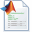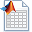## matlab initialize函数,未定义函数或变量 'initialization'。_一支援的博客-程序员秘密

tic % 计时

%% 清空环境导入数据

clear

clc

close all

format long

%% GWO-SVR

% 训练/测试数据准备(用前3天预测后一天),用前100天做测试数据

train_input(1,:)=wndspd(1:97);

train_input(2,:)=wndspd(2:98);

train_input(3,:)=wndspd(3:99);

train_output=[wndspd(4:100)]';

test_input(1,:)=wndspd(101:end-3);

test_input(2,:)=wndspd(102:end-2);

test_input(3,:)=wndspd(103:end-1);

test_output=[wndspd(104:end)]';

[input_train,rule1]=mapminmax(train_input);

[output_train,rule2]=mapminmax(train_output);

input_test=mapminmax('apply',test_input,rule1);

output_test=mapminmax('apply',test_output,rule2);

%% 利用灰狼算法选择最佳的SVR参数

SearchAgents_no=20; % 狼群数量

Max_iteration=20; % 最大迭代次数

dim=2; % 此例需要优化两个参数c和g

lb=[0.01,0.01]; % 参数取值下界

ub=[100,100]; % 参数取值上界

Alpha_pos=zeros(1,dim); % 初始化Alpha狼的位置

Alpha_score=inf; % 初始化Alpha狼的目标函数值，change this to -inf for maximization problems

Beta_pos=zeros(1,dim); % 初始化Beta狼的位置

Beta_score=inf; % 初始化Beta狼的目标函数值，change this to -inf for maximization problems

Delta_pos=zeros(1,dim); % 初始化Delta狼的位置

Delta_score=inf; % 初始化Delta狼的目标函数值，change this to -inf for maximization problems

Positions=initialization(SearchAgents_no,dim,ub,lb);

Convergence_curve=zeros(1,Max_iteration);

l=0; % 循环计数器

while l

for i=1:size(Positions,1)  % 遍历每个狼

% 若搜索位置超过了搜索空间，需要重新回到搜索空间

Flag4ub=Positions(i,:)>ub;

Flag4lb=Positions(i,:)

% 若狼的位置在最大值和最小值之间，则位置不需要调整，若超出最大值，最回到最大值边界；

% 若超出最小值，最回答最小值边界

Positions(i,:)=(Positions(i,:).*(~(Flag4ub+Flag4lb)))+ub.*Flag4ub+lb.*Flag4lb; % ~表示取反

% 计算适应度函数值

cmd = ['-s 3 -t 2',' -c ',num2str(Positions(i,1)),' -g ',num2str(Positions(i,2))];

model=svmtrain(output_train',input_train',cmd); % SVM模型训练

[~,fitness]=svmpredict(output_test',input_test',model); % SVM模型预测及其精度

fitness=fitness(2); % 以平均均方误差MSE作为优化的目标函数值

if fitness

Alpha_score=fitness; % 则将Alpha狼的目标函数值更新为最优目标函数值

Alpha_pos=Positions(i,:); % 同时将Alpha狼的位置更新为最优位置

end

if fitness>Alpha_score && fitness

Beta_score=fitness; % 则将Beta狼的目标函数值更新为最优目标函数值

Beta_pos=Positions(i,:); % 同时更新Beta狼的位置

end

if fitness>Alpha_score && fitness>Beta_score && fitness

Delta_score=fitness; % 则将Delta狼的目标函数值更新为最优目标函数值

Delta_pos=Positions(i,:); % 同时更新Delta狼的位置

end

end

a=2-l*((2)/Max_iteration); % 对每一次迭代，计算相应的a值，a decreases linearly fron 2 to 0

for i=1:size(Positions,1) % 遍历每个狼

for j=1:size(Positions,2) % 遍历每个维度

% 包围猎物，位置更新

r1=rand(); % r1 is a random number in [0,1]

r2=rand(); % r2 is a random number in [0,1]

A1=2*a*r1-a; % 计算系数A，Equation (3.3)

C1=2*r2; % 计算系数C，Equation (3.4)

% Alpha狼位置更新

D_alpha=abs(C1*Alpha_pos(j)-Positions(i,j)); % Equation (3.5)-part 1

X1=Alpha_pos(j)-A1*D_alpha; % Equation (3.6)-part 1

r1=rand();

r2=rand();

A2=2*a*r1-a; % 计算系数A，Equation (3.3)

C2=2*r2; % 计算系数C，Equation (3.4)

% Beta狼位置更新

D_beta=abs(C2*Beta_pos(j)-Positions(i,j)); % Equation (3.5)-part 2

X2=Beta_pos(j)-A2*D_beta; % Equation (3.6)-part 2

r1=rand();

r2=rand();

A3=2*a*r1-a; % 计算系数A，Equation (3.3)

C3=2*r2; % 计算系数C，Equation (3.4)

% Delta狼位置更新

D_delta=abs(C3*Delta_pos(j)-Positions(i,j)); % Equation (3.5)-part 3

X3=Delta_pos(j)-A3*D_delta; % Equation (3.5)-part 3

% 位置更新

Positions(i,j)=(X1+X2+X3)/3;% Equation (3.7)

end

end

l=l+1;

Convergence_curve(l)=Alpha_score;

end

bestc=Alpha_pos(1,1);

bestg=Alpha_pos(1,2);

bestGWOaccuarcy=Alpha_score;

%% 打印参数选择结果

disp('打印选择结果');

str=sprintf('Best Cross Validation Accuracy = %g%%，Best bestc = %g，Best bestg = %g',bestGWOaccuarcy*100,bestc,bestg);

disp(str)

%% 利用回归预测分析最佳的参数进行SVM网络训练

cmd_gwo_svr=['-s 3 -t 2',' -c ',num2str(bestc),' -g ',num2str(bestg)];

model_gwo_svr=svmtrain(output_train',input_train',cmd_gwo_svr); % SVM模型训练

%% SVM网络回归预测

[output_test_pre,acc]=svmpredict(output_test',input_test',model_gwo_svr); % SVM模型预测及其精度

test_pre=mapminmax('reverse',output_test_pre',rule2);

test_pre = test_pre';

err_pre=wndspd(104:end)-test_pre;

figure('Name','测试数据残差图')

set(gcf,'unit','centimeters','position',[0.5,5,30,5])

plot(err_pre,'*-');

figure('Name','原始-预测图')

plot(test_pre,'*r-');hold on;plot(wndspd(104:end),'bo-');

legend('预测','原始')

set(gcf,'unit','centimeters','position',[0.5,13,30,5])

result=[wndspd(104:end),test_pre]

MAE=mymae(wndspd(104:end),test_pre)

MSE=mymse(wndspd(104:end),test_pre)

MAPE=mymape(wndspd(104:end),test_pre)

%% 显示程序运行时间

toc2019-6-17 13:46 上传

70 Bytes, 下载次数: 412019-6-17 13:46 上传

85 Bytes, 下载次数: 332019-6-17 13:46 上传

74 Bytes, 下载次数: 362019-6-17 13:46 上传

1.8 KB, 下载次数: 662019-6-17 13:46 上传

19.7 KB, 下载次数: 412019-6-17 13:46 上传

447 Bytes, 下载次数: 35

### 智能推荐

MongoDB环境配置及NodeJS使用MongoDB

### SystemWeaver — 电子电气协同设计研发平台_经纬恒润的博客-程序员秘密

SystemWeaver — 电子电气协同设计研发平台当前电子电气系统在汽车领域的应用广泛，系统的复杂性、关联性日益提升。其设计整合了多门工程学科，需要提供面向软件、硬件、网络、电气等多领域交织而导致的复杂系统解决方案。并且，随着功能安全、AUTOSAR、SOA、以太网通讯等新要求、方法、概念的提出，主机厂和供应商将面临多方面的严峻挑战。如何实现汽车电子电气平台化设计、定义优化系统架构、实现多领域协同开发，完成新老技术的快速更替和融合将成为未来竞争的核心要素。当前，国内外众多主机厂均着手于通过工具构建产品

### eclipse快捷键大全_weixin_30590285的博客-程序员秘密

eclipse常用快捷键Eclipse的编辑功能非常强大，掌握了Eclipse快捷键功能，能够大大提高开发效率。Eclipse中有如下一些和编辑相关的快捷键。 1. 【ALT+/】Sysout+ System.out.println()Main+[enter]+public static void main(String[] args) 此快捷键为...

### 【Android】自定义相机的实现（支持连续拍照、前后摄像头切换、连续对焦）_android摄像头多次切换怎么做好复用_lankton的博客-程序员秘密

~转载请注明http://blog.csdn.net/u013015161/article/details/46921257介绍这几天，写了一个自定义照相机的demo，支持连续拍照和摄像头切换。由于自己以前没接触过相关的编程，也算是一个学习的过程，在这里做一下记录，同时也分享出来，并附上源码和工程。 效果如图： 左上角switch切换摄像头，右边snap按钮进行拍照。一般流程Android进